American Journal of Aerospace Engineering
Volume 4, Issue 1, February 2017, Pages: 1-5

Mathematical Model of the Liquid Film Flow on the Flat Surface

N. I. Klyuev

Department of Mathematical Modeling in Mechanics, Samara State University, Samara, Russia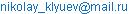N. I. Klyuev. Mathematical Model of the Liquid Film Flow on the Flat Surface. American Journal of Aerospace Engineering. Vol. 4, No. 1, 2017, pp. 1-5. doi: 10.11648/j.ajae.20170401.11

Received: December 28, 2016; Accepted: January 19, 2017; Published: February 21, 2017

Abstract: A liquid film on surface of a body decreases frictional resistance and can be used as a boundary layer control element. This article contains a mathematical model of a film flow over a half-plane, directed at an angle to the horizon. Liquid flow depends on gravity and friction with the external air flow. A model of incompressible viscous liquid near the boundary layer is used as the flow model. Summands of motion equation are averaged over the film thickness by the Leibniz rule. The square low is assumed for distribution of longitudinal velocity in the cross-section of the film with regard to the friction at the film's surface. An approximate solution of the problem is received as power series in powers of small parameter. The results are presented in a form diagrams of the film thickness and the average longitudinal velocity over the length of the plate. The mathematical flow model can be used to define flat film flow performance.

Keywords: Film, Liquid, Boundary Layer, Flow, Friction, Small Parameter

Contents

1. Introduction

Liquid film flow problems are quite frequent for technical appendixes and works by many authors. For example, work  presents film research findings for a two-phase gas-liquid flow in short horizontal pipes. The obtained results are compared to the experimental data. Work  contains film flow dynamic properties under a counter current airflow. The design data meet the experiment.

Work  examines a three-dimensional dynamic model of film flow over a cylindrical surface. The film is exposed to gravity, and surface-tension, as well as a drag of a countercurrent gas flow. Work  presents solution for related problem for airflow over a flat plate with a thin liquid film at the surface. The film is formed by extruding the liquid through a porous wall. In work  the film is formed out of the environment (for example, rain drops). The impact of raindrops to the boundary layer is ignored. The mathematical flow model is developed for a zero approximation. Works  -  contain findings of theoretical and numerical research of liquid films, works  –  present experimental research.

Work  presents a model of a thin film flow over an inclined plane. Summands of the Navier-Stokes equation and the energy equation are averaged over the depth of the liquid. Work  proposes a mathematical model for evaporation of a thin film. Work  contains experimental results and numerical simulation of a film flow over a cooled wall. The measurements were done at a flat plate. Work  analytically investigates a condensate film stability problem on a vertical surface. Work  presents an analytical model for a laminar flow of a condensate film over an external surface of an isothermal vertical pipe. Regardless of a big number of works on the flow of liquid films, the interest to this subject does not wane.

The purpose of this research is the development of a mathematical model of a liquid film flow over a flat surface, forced by gravitation and the external airflow for the further simulation of a variable thickness film wave flow.

2. Statement of Problem

Flying vehicles are operated in various climatic zones and under different weather conditions. It is not rare when a liquid film is formed on the surface of a flying vehicle. The occurrence of the film can be caused by atmospheric precipitation; a liquid on the surface of a vehicle can form as a result of condensation of humidity from the surrounding air or artificially, through squeezing-out from a porous wall. During the flight of a flying vehicle the film influences the air boundary layer, thus the velocity field is restructured. In addition, already with comparatively small Reynolds numbers calculated according to the characteristics of the film, waves appear on the surface of the film. Thus, a smooth film reduces the friction resistance, whereas a wavy film increases the friction resistance. It should also be noted that the film may be used as means to control the boundary layer of the body. In any case the film changes the aerodynamic characteristics of the structural elements. That is why a liquid film should be taken into account in the flight of a flying vehicle.

Strictly speaking, the problem of body flow with film is a conjugate problem. Its solution poses considerable difficulties. It is necessary to calculate the characteristics of a boundary layer and a liquid film, which are influencing each other. As a rule, such problems are solved numerically. For an analytical solution of such problem an iterative process can be used. The iterative process consists from an exterior and an interior problem. The exterior problem contains a problem an air boundary layer with a movable wall (the thickness of a film is, as a rule, is neglected). The interior problem is a problem of a liquid film flow with friction on the interfacial area. The friction is determined by the solution of the exterior problem. The velocity of slippage on the wall, for the exterior problem, is determined as the longitudinal liquid velocity on the film surface. The solution process consists in successive solutions of the exterior and interior problems. The solution continues until as the friction and velocity on the film surface will not within the prescribed accuracy.

The proposed procedure assumes presence mathematical models for the exterior and interior problems. In this paper we will discuss the mathematical model of flow of a liquid film on a flat surface under the effect of gravity and interfacial friction. As a surface element we will take a flat plate that moves in immovable air with an angle to the horizon. By reversing motion, we will receive the homogeneous airflow around the plate parallel to the plane of the plate. The liquid enters the film evenly through the porous surface of the plate (Figure 1).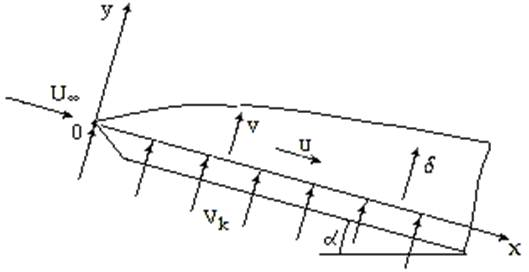Figure 1. Film flow diagram: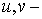the components of the velocity vector,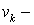velocity of injection mass,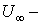velocity of the incoming flow,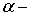angle to the horizontal plate,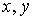- coordinate axis.

The research is limited to a steady flow of the liquid in a variable film forced by gravity and a drag of a countercurrent airflow. Mathematical target setting includes motion equations for the near-the-boundary layer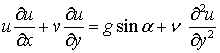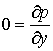(1)

and continuity equation for an incompressible liquid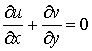(2)

Boundary conditions at the wall are as follows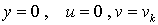(3)

friction with the external airflow is set for the surface of the film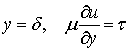(4)

where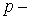pressure,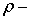density,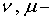kinematic and dynamic viscosity, g – acceleration of gravity,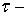friction,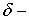film thickness.

3. Method of Solution

The motion equations is completed with a kinematic condition for the film surface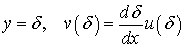(5)

Let's combine the motion and the continuity equations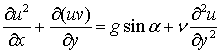(6)

Since the thickness of the film is small enough, viscosity forces will play a major part in the flow development. This case allows adopting the square law for distribution of longitudinal velocity over the film cross-section and using the velocity expression for a uniform thickness film, flowing down along a flat vertical wall with regard to the surface friction(7)

In this case the film thickness and the average longitudinal velocity are the functions of the longitudinal coordinate. Let's average the summands of the equation (6) over the film thickness. For this let's integrate the summands from 0 to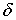in respect of y using the Leibniz rule (derivative of an integral with a variable upper limit)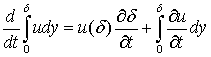there from we will find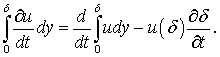The motion equation (6) will change as follows (skew

brackets of the average velocity are hereinafter omitted)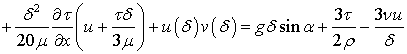(8)

Let's write down the mass conservation equation, for whatlet's integrate the continuity equation (2) from 0 toin

respect of y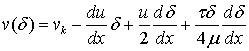(9)

Putting expression (9) into the equation (8), we receive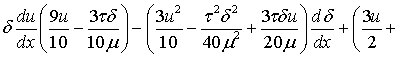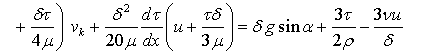(10)

Let's use the film surface kinematic condition (5) and, with regard to the expression (7), write down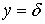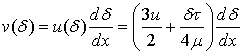(11)

Combination of formulas (9) and (11) will give the mass conservation law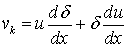(12)

Let's transform the motion equation (10) and the continuity equation (12) with the use of dimensionless variables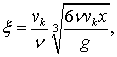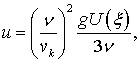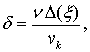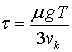(13)

Were performed some transformation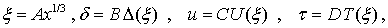where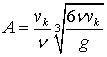,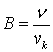,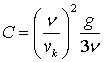.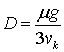,

then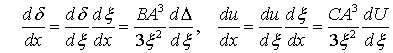.

Dimensionless motion equation will be as follows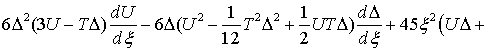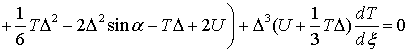(14)

Putting dimensionless variables into the mass conservation equation (12) gives the second differential equation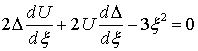(15)

and the initial conditions of the problem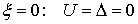(16)

Solving this problem requires setting the film surface friction. Let's omit the film thickness and study the Blasius problem of streamline of a plate with forward air. When moving from the edge of the plate the surface friction becomes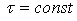. Therefore let's assume the friction value, that is a sufficient approximation if the mass is injected at a distance from the edge of the plate. The value of friction at the film-air boundary is set as an average of friction from the Blasius.

Input data of the problem. Let a flat horizontal plate with the length of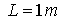is blown over by airflow with velocity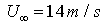at temperature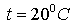. The Reynolds number for a forward air flow is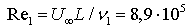, where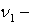the air kinematic viscosity. Let's take the mass injection velocity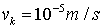, then the dimensionless coordinate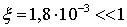. Therefore, solution of (14) and (15) nonlinear differential equations can be presented as rows by the small parameter power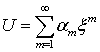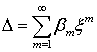(17)

where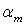and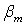(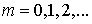) coefficients are defined at a fixed angle of the plate and the given friction value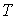. Let's write down the solution for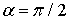and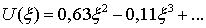(18)

4. Results of the Solution and Discussion

The results of the solution are presented in dimensional way at diagrams on figure 2 and figure 3 forand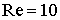, where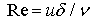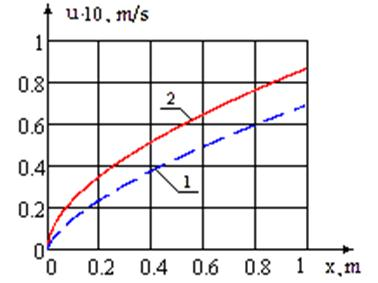Figure 2. Average longitudinal velocity,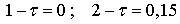.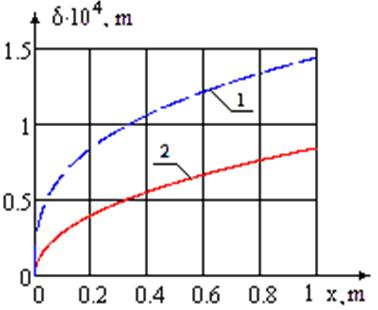Figure 3. Film thickness,As it was anticipated, the concurrent airflow increases the average longitudinal velocity and decreases the film thickness. Diagrams (figure 4 and figure 5) show variation of the average longitudinal speed and the film thickness for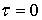and for different plate angles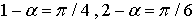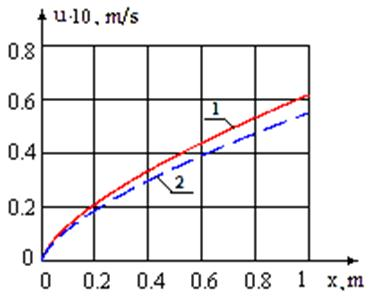Figure 4. Average longitudinal velocity.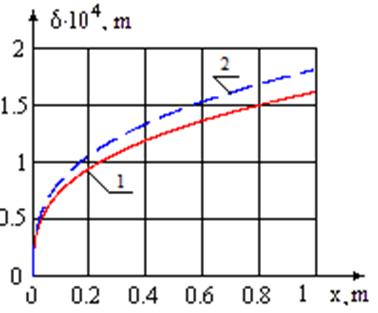Figure 5. Film thickness.

It is seen from the diagrams that increasing the plate angle increases the longitudinal speed and decreases the film thickness. The Reynolds number does not exceed the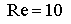. And the film flow is not a wave flow.

5. Conclusion

The mathematical model of a thin liquid layer flow over a flat plate surface allows defining the film properties on the flat elements of an aircraft. The results can be used for calculation of frictional resistance, which is a component of an aircraft resistance. An approximate analytical solution was obtained as a series in terms of small parameter powers taking into account zero and first approximation. The results are presented as graphs of film thickness and average longitudinal velocity depending on the plate length for various angles of slope to the horizon. It is proposed to use the analytical solution of the problem in an iterative process for the conjugate problem, and also as part of the mathematical model of a flow of a wavy film with variable thickness.

References

1. López, J., Pineda, H., Bello, D., Ratkovich, N. Study of liquid-gas two-phase flow in horizontal pipes using high speed filming and computational fluid dynamics. Experimental Thermal and Fluid Science. V.76, 2016, pp.126-134.
2. Lu, H., Lu, L., Luo, Y.,Qi, R. Investigation on the dynamic characteristics of the counter-current flow for liquid desiccant dehumidification. Energy. V.101, 2016, pp. 229-238.
3. Li, M., Lu, Y., Zhang, S., Xiao, Y. A numerical study of effects of counter-current gas flow rate on local hydrodynamic characteristics of falling films over horizontal tubes. Desalination. V.383, 2016, pp. 68-80.
4. Klyuev, N.I., Gimadiev, A. G., Kryukov, Y. A. Two-media boundary layer on a flat plate. IJET. V.6, Issue 5, 2014, pp. 2368-2374.
5. Klyuev, N.I., Kryukov, Y.A. Influence of fluid film on friction of a flat plate. Russian Aeronautics (Iz. VUZ). V.57, Issue 4, 2014, pp.372-377.
6. Camassa, R., Ogrosky, H.R. On viscous film flows coating the interior of a tube: Thin-film and long-wave models. Journal of Fluid Mechanics, V.772, 2015, pp.569-599.
7. Muramatsu, K., Youn, Y., Han, Y.,Hasegawa, Y., Shikazono, N. Numerical study on the effect of initial flow velocity on liquid film thickness of accelerated slug flow in a micro. International Journal of Heat and Fluid Flow. V.54, 2015, pp.77-86.
8. Han, Y., Kanno, H., Ahn, Y.-J., Shikazono, N. Measurement of liquid film thickness in micro tube annular flow. International Journal of Multiphase Flow. V.73, 2015, pp.264-274.
9. Youn, Y.J., Muramatsu, K., Han, Y., Shikazono, N. The effect of initial flow velocity on the liquid film thickness in micro tube accelerated slug flow. International Journal of Multiphase Flow. V.73, 2015, pp.108-117.
10. Ju, P., Brooks, C.S., Ishii, M., Liu, Y., Hibiki, T. Film thickness of vertical upward co- current adiabatic flow in pipes. International Journal of Heat and Mass Transfer. V.89, 2015, pp. 985-995.
11. Richard, G.L., Ruyer-Quil, C., Vila, J.P.  A three-equation model for thin films down an inclined plane. Journal of Fluid Mechanics. V.804, 2016, pp.162-200.
12. Akkuş, Y., Dursunkaya, Z. International Journal of Heat and Mass Transfer. V.101, 2016, pp.742-748.
13. Liu, C.L., Liu, J.L., >Zhu, H.R., Wu, A.S., He, Y.H., Zhou, Z.X. Film cooling sensitivity of laidback fanshape holes to variations in exit configuration and mainstream turbulence intensity. International Journal of Heat and Mass Transfer. V.89, Issue 12145, 2015, pp.1141-1154.
14. Degan, G., Sanya, A., Akowanou, C. Laminar film condensation along a vertical plate embedded in an anisotropic porous medium with oblique principal axes. Heat and Mass Transfer. V.52, Issue10, 2016, pp.2119-2128.
15. Kim, S., Lee, Y.G., Jerng, D.W. Laminar film condensation of saturated vapor on an isothermal vertical cylinder. International Journal of Heat and Mass Transfer. V.83, 2015, pp. 545-551.

 Contents 1. 2. 3. 4. 5.
Article ToolsAbstractPDF(639K)